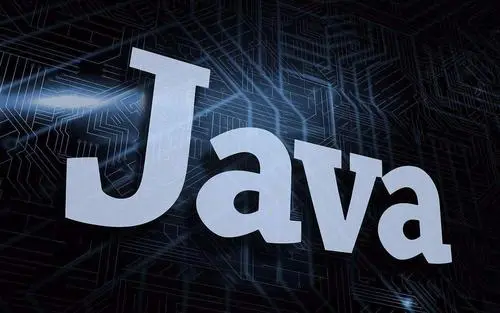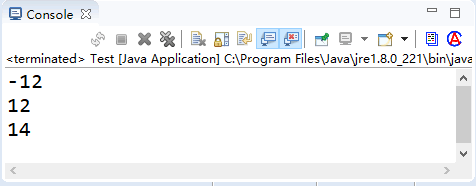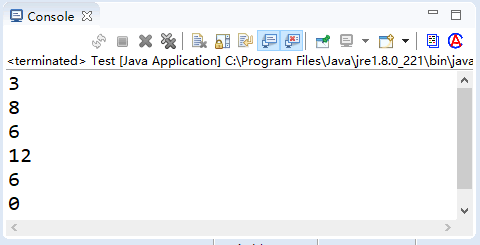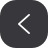# Java学习之算术运算符image.png

Java 中的算术运算符主要用来组织数值类型数据的算术运算，按照参加运算的操作数的不同可以分为一元运算符和二元运算符。

## 一元运算符

-取反符号取反运算b=-a
++自加一先取值再加一，或先加一再取值a++ 或 ++a
--自减一先取值再减一，或先减一再取值a-- 或 --a

• -a 是对 a 取反运算
• a++ 或 a-- 是在表达式运算完后，再给 a 加一或减一
• ++a 或 --a 是先给 a 加一或减一，然后再进行表达式运算

int a = 12;
System.out.println(-a);
int b = a++;
System.out.println(b);
b = ++a;
System.out.println(b);image.pngimage.png

## 二元运算符

+求 a 加 b 的和，还可用于 String 类型，进行字符串连接操作a + b
-求 a 减 b 的差a - b
*求 a 乘以 b 的积a * b
/求 a 除以 b 的商a / b
%取余求 a 除以 b 的余数a % b

int a = 4, b = 2, c = 3;
int d = a * (b + c) % c;

1. int x=2,y=1;
表达式 y/x 的结果是 0。(int是整数，去掉小数部分)
2. float x=2.0f; int y=1;
表达式 y/x 的结果是 0.5。image.png

#### 代码练习

public static void main(String[] args) {
float f1 = 9 % 4;// 保存取余后浮点类型的结果
double da = 9 + 4.5; // 双精度加法
double db = 9 - 3.0; // 双精度减法
double dc = 9 * 2.5; // 双精度乘法
double dd = 9 / 3.0; // 双精度除法
double de = 9 % 4; // 双精度取余
System.out.println("整数的算术运算"); // 整数的加、减、乘、除和取余
System.out.printf("9+4=%d \n", 9 + 4);
System.out.printf("9-4=%d \n", 9 - 4);
System.out.printf("9*4=%d \n", 9 * 4);
System.out.printf("9/4=%d \n", 9 / 4);
System.out.printf("9%%4=%d \n", 9 % 4);
System.out.println("\n浮点数的算术运算"); // 浮点数的加、减、乘、除和取余
System.out.printf("9+4.5f=%f \n", 9 + 4.5f);
System.out.printf("9-3.0f=%f \n", 9 - 3.0f);
System.out.printf("9*2.5f=%f \n", 9 * 2.5f);
System.out.printf("9/3.0f=%f \n", 9 / 3.0f);
System.out.printf("9%%4=%f \n", f1);
System.out.println("\n双精度数的算术运算"); // 双精度数的加、减、乘、除和取余
System.out.printf("9+4.5=%4.16f \n", da);
System.out.printf("9-3.0=%4.16f \n", db);
System.out.printf("9*2.5=%4.16f \n", dc);
System.out.printf("9/3.0=%4.16f \n", dd);
System.out.printf("9%%4=%4.16f \n", de);
System.out.println("\n字符的算术运算"); // 对字符的加法和减法
System.out.printf("'A'+32=%d \n", 'A' + 32);
System.out.printf("'A'+32=%c \n", 'A' + 32);
System.out.printf("'a'-'B'=%d \n", 'a' - 'B');
}

整数的算术运算
9+4=13
9-4=5
9*4=36
9/4=2
9%4=1

9+4.5f=13.500000
9-3.0f=6.000000
9*2.5f=22.500000
9/3.0f=3.000000
9%4=1.000000

9+4.5=13.5000000000000000
9-3.0=6.0000000000000000
9*2.5=22.5000000000000000
9/3.0=3.0000000000000000
9%4=1.0000000000000000

'A'+32=97
'A'+32=a
'a'-'B'=31

## 算术赋值运算符

+=加赋值a += b、a += b+3
-=减赋值a -= b
*=乘赋值a *= b
/=除赋值a /= b
%=取余赋值a %= b

int a = 1;
int b = 2;
a += b; // 相当于 a = a + b
System.out.println(a);
a += b + 3; // 相当于 a = a + b + 3
System.out.println(a);
a -= b; // 相当于 a = a - b
System.out.println(a);
a *= b; // 相当于 a=a*b
System.out.println(a);
a /= b; // 相当于 a=a/b
System.out.println(a);
a %= b; // 相当于 a=a%b
System.out.println(a);image.pngimage.png
07-11
11-17374
02-2876
07-10179
01-14596
03-061037
02-12187
04-26325
02-03327
07-13142
02-21248
06-13201
02-1279
03-03929
04-2058
07-25324
03-10329
10-1391
04-25167

### “相关推荐”对你有帮助么？

•非常没帮助
•没帮助
•一般
•有帮助
•非常有帮助

©️2022 CSDN 皮肤主题：鲸 设计师：meimeiellie被折叠的  条评论 为什么被折叠?到【灌水乐园】发言Lee_5566

¥2 ¥4 ¥6 ¥10 ¥20余额支付 (余额：-- )扫码支付获取中扫码支付点击重新获取扫码支付1.余额是钱包充值的虚拟货币，按照1:1的比例进行支付金额的抵扣。
2.余额无法直接购买下载，可以购买VIP、C币套餐、付费专栏及课程。余额充值